https://doi.org/10.5194/amt-16-3715-2023
https://doi.org/10.5194/amt-16-3715-2023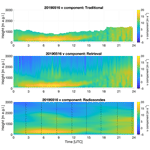# Using optimal estimation to retrieve winds from velocity-azimuth display (VAD) scans by a Doppler lidar

Sunil Baidar, Timothy J. Wagner, David D. Turner, and W. Alan Brewer
Abstract

Share
Dates
1 Introduction and background

The kinematic profile of the planetary boundary layer (PBL) has a significant impact on disciplines throughout the atmospheric sciences. Low-level wind shear can determine storm mode (e.g., Davies and Johns, 1993) and has significant impacts on aviation safety (e.g., Gultepe et al., 2019; Thobois et al., 2018), while knowledge of the wind profile within the PBL is a significant factor in siting wind energy installations (Banta et al., 2013). High-temporal-resolution observations of the wind profile are crucial for understanding numerous atmospheric processes. While radiosondes remain the standard by which all profiling measuring systems are evaluated, they are not well-suited toward capturing the evolution of boundary layer wind profiles due to their substantial cost per observation and significant time required to prepare and execute each observation. Alternative ways of observing atmospheric wind profiles have been developed, including active remote sensing with radars, lidars, and sodars; passive remote sensing with satellites; and in situ observations with commercial aircraft or uncrewed aircraft systems (UASs).

To address the need for rapid sampling of the wind profile in the PBL, manufacturers have developed low-powered commercial coherent Doppler lidar (CDL) wind profilers. These systems feature turnkey operation, are quick and easy to deploy, and have the ability to run unattended for significant periods of time. Fundamentally, Doppler lidars measure the velocity of scatterers along the emitted beam (radial velocity or line-of-sight velocity, LOSV); it is assumed that the one-dimensional speed of the scatterers is the same as the wind speed along that direction since the primary scatterers are aerosols. Observations of the vertical profile of the horizontal or three-dimensional wind vector can be retrieved from CDL-observed radial velocities using techniques like velocity-azimuth display (VAD) or Doppler beam swinging (DBS). In both of these techniques, lidar measurements along multiple non-coplanar angles are used to reconstruct the vertical profile of the wind vector under the assumption that winds are horizontally homogeneous within the volume observed by the lidar and that they do not evolve during the period (usually a minute or less) in which a set of scans is collected. Numerous studies comparing Doppler lidar wind profile retrievals to in situ observations from radiosondes or instrumented towers and masts have shown that CDLs are a reliable and effective way of measuring wind profiles in the PBL (e.g., Choukulkar et al., 2017; Klein et al., 2015).

While the theoretical maximum range of CDLs is 10 km or more and is only limited by the pulse repetition frequency of the emitter and the number of range gates in the detection system, the need for the signal to be scattered and returned to the lidar means that the effective range is much less. CDLs usually feature a laser emitting at 1.5 µm. This wavelength is short enough to be sensitive to aerosols, cloud droplets, and some precipitation but not so short that it is significantly impacted by molecular scattering. This means it can be a challenge to obtain wind observations at times and heights where aerosol content is low, such as above the top of the PBL. In practice, CDL-observed wind profiles usually extend to 1–2 km above ground level (a.g.l.). While this observation depth is more than sufficient for wind energy applications, other processes such as PBL entrainment or mesoscale dynamics extend to higher altitudes and are difficult to assess with operational CDL retrievals. Furthermore, since the aerosol concentration is not constant, the maximum effective height of CDL-observed wind profiles varies substantially throughout the day and from one day to the next. This makes it challenging to develop continuous products that rely on CDL profiles as the valid range is constantly changing. Various techniques have been developed to extend the range of wind profiles from scanning CDL, including accumulation of signal power spectra estimates for direct estimation of the wind vector without estimating radial wind velocities for individual azimuth angles (Smalikho, 2003; Stephan et al., 2019). Although these advanced techniques are able to extract information from noisier Doppler spectra, they are still limited by the availability of the scatterers and, hence, do not provide consistent vertical coverage.

2 Methodology

$\begin{array}{}\text{(1)}& {\mathbit{\psi }}^{\mathrm{2}}=\sum _{i=\mathrm{1}}^{N}\frac{{\left({\mathbit{x}}_{r}{\mathbf{f}}_{i}^{\mathrm{T}}-{\mathbit{y}}_{ri}\right)}^{\mathrm{2}}}{{\mathit{\sigma }}_{ri}^{\mathrm{2}}},\end{array}$

with ${\mathbf{f}}_{i}=\left[\mathrm{sin}{\mathit{\theta }}_{i}\cdot \mathrm{cos}\mathit{\alpha },\mathrm{cos}{\mathit{\theta }}_{i}\cdot \mathrm{cos}\mathit{\alpha },\mathrm{sin}\mathit{\alpha }\right]$ representing the measurement geometry of individual beams.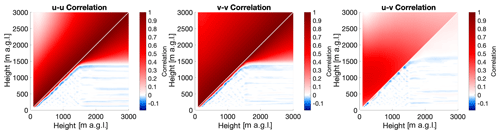Figure 1Correlation matrices for uu (a), vv (b), and uv (c) for the month of July. The upper-left half of each panel shows the correlation of the a priori profile, while the lower-right half shows the correlation of the posterior profile for a clear-sky retrieval at 02:35 UTC on 16 July 2019.

One way of integrating the level-to-level correlations with CDL radial velocity observations to produce continuous wind profiles is through the implementation of an optimal estimation retrieval (OE; Rodgers, 2000). In optimal estimation, a set of measurements y is related to the state vector x, which contains parameters describing the current atmospheric state, by a forward model F:

$\begin{array}{}\text{(2)}& \mathbit{y}=\mathbf{F}\left(\mathbit{x},b\right)+\mathit{ϵ},\end{array}$

where b represents model parameters that are not retrieved, and ϵ represents the model error. In essence, the forward model maps the state of the atmosphere to a set of variables that can be observed directly and contains the physical and instrumental factors that describe the measurements. For many remote sensing applications, the forward model is a radiative transfer model that converts the state of the atmosphere (such as profiles of temperature, water vapor, and trace gases) to radiances at various wavelengths measured by satellites or ground-based radiometers. Through the optimal estimation technique, this relationship is inverted, so a set of observations can be used to obtain the atmospheric state. The optimal estimation technique has been extensively used for retrievals of atmospheric constituent profiles from passive remote sensing measurements where the problem is generally ill-determined (e.g., Kuang et al., 2002; Maahn et al., 2020; Turner and Blumberg, 2019; Turner and Löhnert, 2014). Since ill-determined problems can produce an infinite number of solutions, a priori information in the form of the mean and covariance of the state vector is used as a constraint to help the algorithm obtain a solution that is both physically possible and statistically likely to occur for a particular location and time of year.

In the present case, in which scanning CDL measurements of radial velocities at different azimuth (θ) and elevation (α) angles are being used to obtain the components of the wind vector (u, v, and w), the forward model is simply the geometry of the measurement that maps the wind vector to the radial coordinate system. It is given by

$\begin{array}{}\text{(3)}& \mathbf{F}=\left[\mathrm{sin}\mathit{\theta }\cdot \mathrm{cos}\mathit{\alpha },\mathrm{cos}\mathit{\theta }\cdot \mathrm{cos}\mathit{\alpha },\mathrm{sin}\mathit{\alpha }\right].\end{array}$

If one assumes that the vertical velocity w is much smaller than the horizontal velocity, then the contributions of w to the radial wind vector can be neglected. The forward model then reduces to

$\begin{array}{}\text{(4)}& \mathbf{F}=\left[\mathrm{sin}\mathit{\theta }\cdot \mathrm{cos}\mathit{\alpha },\mathrm{cos}\mathit{\theta }\cdot \mathrm{cos}\mathit{\alpha }\right].\end{array}$

Since the forward model F is independent of the state vector x, the Jacobian K of the forward model F with respect to the elements of the state vector $\mathbit{x}=\left[\mathbit{u},\mathbit{v}\right]$ is also the forward model

$\begin{array}{}\text{(5)}& \mathbf{K}=\frac{\mathrm{d}\mathbf{F}}{\mathrm{d}\mathbit{x}}=\mathbf{F}.\end{array}$

Equation (2) can then be linearized as

$\begin{array}{}\text{(6)}& \mathbit{y}=\mathbf{K}\mathbit{x}+\mathit{ϵ}.\end{array}$

The maximum a posteriori solution for Eq. (6) is

$\begin{array}{}\text{(7)}& \mathbit{x}={\mathbit{x}}_{\mathrm{a}}+{\left({\mathbf{K}}^{\mathbf{T}}{\mathbf{S}}_{\mathit{ϵ}}^{-\mathrm{1}}\mathbf{K}+{\mathbf{S}}_{\mathrm{a}}^{-\mathrm{1}}\right)}^{-\mathrm{1}}{\mathbf{K}}^{\mathbf{T}}{\mathbf{S}}_{\mathit{ϵ}}^{-\mathrm{1}}\left(\mathbit{y}-\mathbf{K}{\mathbit{x}}_{\mathrm{a}}\right),\end{array}$

where xa is the a priori profile, and Sa and Sϵ are the a priori and measurement error covariance matrices, respectively. The VADoe retrievals are performed on a fixed vertical resolution defined by the range gate size of the DL measurement. Note that Eq. (5) has an analytical solution, which is the VADtrad result, but provides an unreasonable solution when the measurement SNR is low. This is the reason the VADtrad algorithm is performed layer by layer and an SNR threshold is applied. Since the present work evaluates the VADoe retrieval at the ARM SGP Central Facility, the a priori information is calculated from 15 years of profiles of wind speed and direction observed by radiosondes launched at that site to create monthly mean u and v profiles (xa) and covariances (Sa). By using monthly a priori information instead of a single priori dataset that spans all seasons for all retrievals, natural variation in the winds can be captured. Few locations will have the in situ observational density that the ARM SGP site does, but alternate sources of a priori data could include Airborne Meteorological Data Relay (AMDAR) (Moninger et al., 2003) observations or model output.

Radial velocity uncertainty (σr) for a range gate is estimated by calculating the mean of the variance of radial velocity over two neighboring range gates for each azimuthal stare. For a VAD scan with n azimuth angles, the radial velocity uncertainty for the jth range gate is given by

$\begin{array}{}\text{(8)}& {\mathit{\sigma }}_{\mathrm{r}}^{\mathrm{2}}\left({r}_{j}\right)=\frac{\mathrm{1}}{\mathrm{3}n}\sum _{i=\mathrm{1}}^{n}\sum _{k=j-\mathrm{1}}^{j+\mathrm{1}}{\left({\mathbit{y}}_{\mathrm{r}}\left({\mathit{\theta }}_{i},{r}_{k}\right)-\stackrel{\mathrm{‾}}{{\mathbit{y}}_{\mathrm{r}}}\left({\mathit{\theta }}_{i},{r}_{j}\right)\right)}^{\mathrm{2}},\end{array}$

where

$\begin{array}{}\text{(9)}& \stackrel{\mathrm{‾}}{{\mathbit{y}}_{\mathrm{r}}}\left({\mathit{\theta }}_{i},{r}_{j}\right)=\frac{\mathrm{1}}{\mathrm{3}}\sum _{k=j-\mathrm{1}}^{j+\mathrm{1}}{\mathbit{y}}_{\mathrm{r}}\left({\mathit{\theta }}_{i},{r}_{k}\right).\end{array}$

This formulation is similar to the Trial 2 radial velocity uncertainty formulation given in Newsom et al. (2017), where radial velocity uncertainty is calculated over consecutive scans and neighboring range gates. This formulation was found to result in the best agreement between wind speed and direction precision estimates from the VADtrad algorithm and sonic anemometer measurements from the collocated 300 m tower at the Boulder Atmospheric Observatory during the eXperimental Planetary boundary-layer Instrument Assessment (XPIA) field campaign (Lundquist et al., 2017). Unlike Newsom et al. (2017), the formulation given by Eq. (8) assumes isotropy in atmospheric variance for a given range gate. Because Eq. (8) assumes isotropy and ignores SNR dependency of measurement uncertainty, the instrument noise component σn is added to σr to compute total measurement error. Figure 2 shows the CDL radial velocity precision as a function of the SNR determined from ARM SGP C1 Doppler lidar vertical stare measurements using the method described in Lenschow et al. (2000) and available as part of the standard ARM vertical velocity statistics dataset (Newsom et al., 2019a).

The total measurement error variance σϵ for a given viewing geometry i used for constructing the measurement error covariance matrix is then given by

$\begin{array}{}\text{(10)}& {\mathit{\sigma }}_{{\mathit{ϵ}}_{i}}^{\mathrm{2}}={\mathit{\sigma }}_{\mathrm{r}}^{\mathrm{2}}+{\mathit{\sigma }}_{{n}_{i}}^{\mathrm{2}}.\end{array}$

Both the maximum possible σr and σn are limited by the CDL measurement bandwidth (±19.4 m s−1 for the SGP lidar) and are much smaller in magnitude than the variability described by the a priori covariance (see Fig. 1). This results in the measurement being artificially weighted higher, even when the measurement has little to no information (region to the left of the dashed vertical line in Fig. 2). In order to overcome this, σn values are set to a large number (100 m s−1) for an SNR below 0.005 ($\sim -\mathrm{23}$ dB). This value needs to be optimized depending upon the number of azimuth beams used in the retrieval. The non-diagonal elements were set to 0, assuming there was no correlation between the uncertainties at different range gates and different azimuth angles.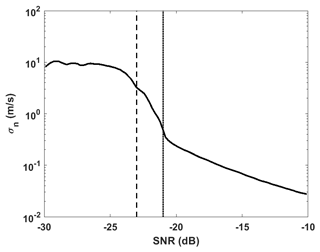Figure 2Measurement precision as a function of the SNR for a day showing different SNR thresholds applied for VADtrad (dotted line) and VADoe retrievals (dashed line).

The solution given by Eq. (7) is a weighted mean of the a priori profile and the information from the measurement. The weight is given by the averaging kernel matrix A,

$\begin{array}{}\text{(11)}& \mathbf{A}={\left({\mathbf{K}}^{\mathbf{T}}{\mathbf{S}}_{\mathit{ϵ}}^{-\mathrm{1}}\mathbf{K}+\phantom{\rule{0.125em}{0ex}}{\mathbf{S}}_{\mathrm{a}}^{-\mathrm{1}}\right)}^{-\mathrm{1}}{\mathbf{K}}^{\mathbf{T}}{\mathbf{S}}_{\mathit{ϵ}}^{-\mathrm{1}}\mathbf{K}.\end{array}$

The retrieval at any range gate is an average of the whole profile weighted by the row of the averaging kernel matrix corresponding to that range gate. The A matrix also can be used to determine the number of independent pieces of information retrieved (often quantified as the degrees of freedom, or DOF) as well as an estimate of the vertical resolution of the retrieved profile at a given level. Note that in a traditional VAD level-by-level retrieval, each range gate is considered independent, and the range gate resolution defines the vertical resolution of retrieved profiles. For an ideal retrieval scenario, A is the identity matrix, the DOF equal the number of retrieved profile layers, and the averaging kernels peak at their corresponding altitudes. In reality, the retrieved profile is a smoothed version of the true profile. In the case of the scanning CDL measurements, A is close to an identity matrix throughout most of the PBL where the SNR is relatively high. As a result, the a priori profile provides minimal to no constraint at those altitudes. However, at altitudes where the measurement SNR is low, the VADoe retrieval is capable of providing an a priori-constrained retrieval, even below the SNR threshold usually applied to VAD retrievals. In cases when there are very few or no valid CDL measurements (e.g., a very low aerosol loading, foggy or rainy day), the retrieved profiles are only constrained by the a priori profile and, hence, follow the a priori profile. Such profiles can easily by identified using the A matrix, DOF, or retrieval error.

One of the advantages of the optimal estimation technique is that uncertainties from both the instrument and the retrieval are propagated throughout the process, so an overall error for each individual observation can be easily quantified. The posterior error covariance matrix which includes contributions from smoothing error and measurement error is given by

$\begin{array}{}\text{(12)}& {\mathbf{S}}_{\mathrm{op}}={\left({\mathbf{S}}_{\mathrm{a}}^{-\mathrm{1}}+\phantom{\rule{0.125em}{0ex}}{\mathbf{K}}^{\mathbf{T}}{\mathbf{S}}_{\mathit{ϵ}}^{-\mathrm{1}}\mathbf{K}\right)}^{-\mathrm{1}}.\end{array}$

An additional source of uncertainty is the accuracy of the forward model, which is affected by the assumption of horizontally homogeneous wind flow; the isotropic turbulence and vertical velocity component are negligible. This forward model error is given by

$\begin{array}{}\text{(13)}& {\mathbf{S}}_{\mathrm{f}}={\mathbf{G}}_{y}\mathrm{\Delta }{f}^{\mathrm{2}}{\mathbf{G}}_{y}^{T}={\mathbf{G}}_{y}{\left[f\left(\mathbit{x},b,b{}^{\prime }\right)-\mathbf{F}\left(\mathbit{x},b\right)\right]}^{\mathrm{2}}{\mathbf{G}}_{y}^{T},\end{array}$

where Gy=AK is the gain matrix; f is the idealized forward model, which includes all the correct physics; and F is the simplified approximation. In an ideal scenario, $f\left(\mathbit{x},b,b{}^{\prime }\right)=\mathbit{y}$. Therefore, we calculated the forward model error as

$\begin{array}{}\text{(14)}& {\mathbf{S}}_{\mathrm{f}}={\mathbf{G}}_{y}\mathrm{\Delta }{f}^{\mathrm{2}}{\mathbf{G}}_{y}^{T}={\mathbf{G}}_{y}{\left[\mathbit{y}-\mathbf{K}\mathbit{x}\right]}^{\mathrm{2}}{\mathbf{G}}_{y}^{T}.\end{array}$

The total retrieval error covariance Stotal is given by

$\begin{array}{}\text{(15)}& {\mathbf{S}}_{\mathrm{total}}={\mathbf{S}}_{\mathrm{op}}+{\mathbf{S}}_{\mathrm{f}}.\end{array}$

The square root of the diagonal element of Stotal provides the 1σ uncertainty for the retrieved u and v profiles.

3 Results

ARM operates a total of five CDLs at the SGP site: one Halo Streamline XR at the C1 facility and four Halo Streamline systems (Pearson et al., 2009) at extended facilities that surround the C1 site at a distance of approximately 50 km. Each ARM CDL makes near-continuous measurements of radial velocity and attenuated backscatter coefficient profiles at a wavelength of 1.5 µm. These CDLs are sensitive to aerosols but not molecular backscatter, and hence the measurements are confined to the PBL. Details about the ARM Doppler lidars, their operations, and data products are found in Newsom and Krishnamurthy (2020). We used the CDL measurements at the SGP C1 (ARM, 2010) for this study. The Doppler lidar at the SGP C1 site that is further examined in this work operates with 30 m range gate resolution and 1.3 s time resolution. It is typically configured to perform one eight-beam PPI scan at a 60 elevation angle every 15 min and performs vertical stares during the remaining time. The PPI scan takes approximately 40 s. Horizontal wind profiles are retrieved from the PPI scan using the VAD method. The ARM DL wind retrieval algorithm is described in detail in Newsom et al. (2019b). It employs an SNR threshold of 0.008 (21 dB) to filter out poor-quality radial velocity data before computing wind profiles.

To evaluate the performance of the optimal estimation retrieval against real-world observations, VADoe retrievals from the ARM SGP C1 lidar were processed for the entirety of the 2019 calendar year. Normally, radiosondes are launched from the SGP site four times a day, but the launch frequency was doubled to eight daily sondes for the 3-month period lasting from May through July 2019. In all, over 1600 radiosondes were collocated with Doppler lidar profiles during the year-long study period. Each radiosonde was temporally matched to the Doppler lidar profile that was taken nearest in time to the radiosonde launch time. Radiosondes that were launched more than 30 min from the nearest valid lidar observation were excluded from this analysis.

An important parameter for evaluating the utility of an optimal estimation retrieval is the information content. One measure of this is the degrees of freedom of the signal (DFS) which can be used to identify how many unique pieces of information are present in the retrieval as well as determine at what altitudes the information can be found. While the OE wind retrieval is output onto a fixed grid with 113 evenly spaced levels from the surface to 3000 m, the fact that OE-retrieved observations are overlapping weighted averages of various depths in the atmosphere means that there will be fewer than 113 uncorrelated pieces of information in the output. The cumulative DFS of the retrieval at the nth level are the sum of the first n elements of the diagonal of the averaging kernel A (Eq. 11); the total DFS of the retrieval are thus simply the trace of A. Figure 3 shows the mean and 25th and 75th percentiles of the cumulative DFS as a function of height for the more than 1600 OE wind profiles that were matched to a radiosonde. On average, the total profile DFS are approximately 15, though the variability ranges from 9.5 to 18.5, and the u and v DFS are effectively identical. Most of the DFS are concentrated in the lowest 1000 m, with approximately 10.3 DFS on average below that height, which means the true vertical resolution of the DL is around 100 m. True vertical resolution of the DL wind profiles can be improved by including multiple PPIs at different EAs and increasing the number of azimuth angles in a PPI scan. However, with roughly 5 DFS in the OE retrieval above 1000 m, the retrieval can still provide valuable information about an otherwise under observed layer of the atmosphere. An advantage of calculating the cumulative DFS and the related true vertical resolution profiles from the optimal estimation retrieval is that it easily facilitates the assimilation of the observed wind profiles into numerical weather prediction (NWP; Coniglio et al., 2019).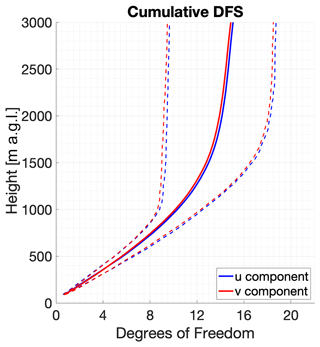Figure 3Vertical profile of the mean (solid) and 25th and 75th percentile (dashed) cumulative degrees of freedom of the signal calculated from the OE wind retrieval for both the u component (blue) and v component (red).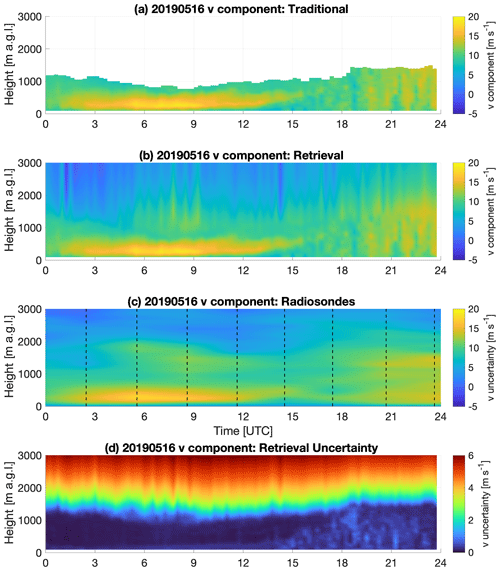Figure 4Time–height cross sections of the v component of the wind on 16 May 2019 as observed by VADtrad (a), VADoe (b), and radiosonde (c). The uncertainty in the VADoe retrieval is shown in panel (d) with a different color scale to enhance detail. Radiosondes were launched every 3 h at the times indicated by dashed lines in the third panel. Radiosonde data are interpolated in time for illustration purposes. Time is in UTC; local time is UTC−5.

To facilitate intercomparisons between the radiosondes and both VADtrad and VADoe, the same vertical grid from the traditional VAD technique was used for the OE output, and the radiosonde observations were averaged to that grid. Quality control measures included rejecting VAD observations with an absolute value greater than 50 m s−1 and OE retrievals where the OE-derived measurement uncertainty exceeded 5 m s−1. Note that due to the stringent SNR threshold ($<-$21 dB) applied to the VADtrad data from the ARM database, there were no VADtrad observations with uncertainty greater than 5 m s−1.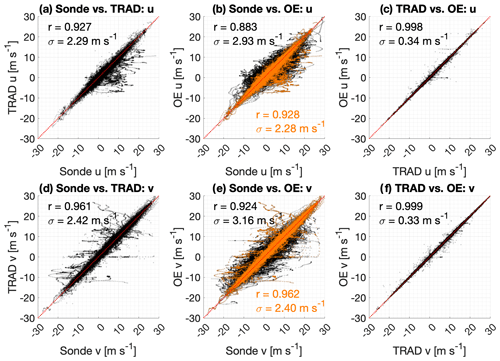## 3.2 Differences as a function of height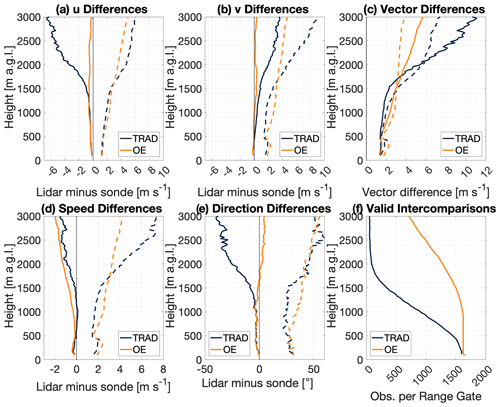Figure 6Vertical profiles of the bias (solid line) and 1σ uncertainty (dashed line) for VADoe (orange) and VADtrad (dark blue) for (a) u winds (m s−1), (b) v winds (m s−1), (c) wind vector differences (m s−1), (d) wind speed (m s−1), and (e) wind direction (). Panel (f) shows the number of valid sonde versus lidar intercomparisons as a function of height for both algorithms.

While the retrieval is conducted in terms of u and v, it is instructive to evaluate how the retrieval performs in terms of wind speed and direction. These are presented in Fig. 6d and e, respectively. The biases for both speed and direction are effectively identical for the two observation sets below 500 m, with a value of approximately 0.5 m s−1. Above that height, the VADoe observations show slightly more negative speed biases than the VADtrad observations do. Again, the speed uncertainty is slightly larger for the VADoe data until 1600 m at which point the VADtrad uncertainty increases rapidly. Direction differences show identical biases of approximately −5 until 1200 m, at which point the VADoe bias becomes less negative and starts to become positive, while the VADtrad bias becomes markedly more negative with height.

Figure 6f also shows the number of valid intercomparisons as a function of height by showing the number of valid lidar–sonde intercomparisons for each lidar range gate. Here, it is clear how rapidly the number of VADtrad observations decreases with height due to the decreasing concentration of scatterers. At 1 km a.g.l., the number of observations is only 59 % of what it was at the lowest range gate. By comparison, the VADoe retrieval still has 98 % of the lowest-level observations. The number of VADoe observations decreases with height due to the imposition of the 5 m s−1 gross error check noted earlier, but it is clear that the decrease in the availability of the VADoe product with increasing height is much less than it is for the VADtrad wind profiles.

## 3.3 Differences as a function of SNR values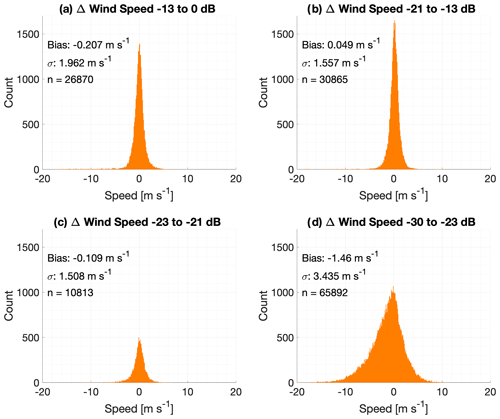Figure 7Histograms of the VADoe retrieval-minus-radiosonde differences in wind speed (m s−1) for four different bands of lidar signal-to-noise ratio.

Figures 7–9d show observations that would not be available for VADtrad retrieval at all. As expected, both the bias and uncertainty are higher for this SNR group. The wind speed (direction) bias and uncertainty of this group of observations are −1.46 m s−1 (9.01) and 3.43 m s−1 (87.2), respectively. The wind vector root-mean-square deviation (RMSD) for this SNR group is 4.3 m s−1. While the VADoe observations in these SNR bands depict larger biases and greater uncertainty than the observations in better SNR bands, the wind speed uncertainty is comparable to the Tropospheric Airborne Meteorological Data Reporting (TAMDAR) system (Wagner and Petersen, 2021). The wind vector RMSD of less than 5 m s−1, which is the error threshold used in the analyses, for this SNR group further supports that the VADoe retrieval errors are representative and can be used to select data to meet different application requirements. For example, VADoe data filtered for greater than 5 m s−1 error would meet the World Meteorological Organization (WMO) threshold requirement for horizontal wind measurements in the free troposphere for global- and high-resolution NWP (WMO, 2022). Considering that only 37.1 % of the total dataset evaluated here has an SNR better than −21 dB, the VADoe technique provides many more usable observations.

4 Discussion

One of the biggest challenges of setting up the VADoe retrieval is appropriately scaling the CDL radial velocity measurement error at low SNRs to provide stable retrievals. The CDL radial velocity measurement error is limited by the measurement bandwidth. For example, the measurement bandwidth for the ARM SGP CDL used here is ±19.4 m s−1. This maximum measurement error is smaller than the a priori error (standard deviation). This becomes even smaller when you consider multiple radial velocities from different azimuths that are included in the retrieval. If the measurement errors are not inflated appropriately, measurements will always be weighted heavily compared to a priori information, which results in unstable retrievals. Thus, the measurement error at low SNR levels needs to be appropriately scaled accounting for number of azimuths and elevation angles included in the retrieval and magnitude of the a priori error.

Successful implementation of VADoe retrieval requires knowledge of the a priori mean profile and covariance. We used radiosonde measurements to create monthly mean profile and covariance. However, radiosonde measurement sites are limited, which limits the applicability of the VADoe retrieval presented here to locations close to radiosonde sites. Future work should include testing using a priori profiles from other sources such as AMDAR and NWPs. This would make VADoe retrieval more widely applicable and also provide high-time-resolution a priori profiles for the retrieval.

5 Summary and conclusion

Coherent Doppler lidars have many research, operational, and commercial applications. Through deployments around the world, they have proven to be reliable and robust instruments that have significantly enhanced our understanding of numerous processes and phenomena. However, since commercially available low-powered CDLs operating at 1.5 µm wavelength are insensitive to molecular scattering and thus must rely on aerosol scattering, the vertical extent of the wind profiles they observe is limited to the planetary boundary layer where aerosol concentrations are greatest. However, many key atmospheric processes are found at or above the top of the boundary layer, which means that many CDLs are unable to observe them with standard algorithms consistently.

Optimal estimation retrievals have significant advantages for data assimilation. With well-characterized uncertainties for each observation and profiles of degrees of freedom of the signal and vertical resolution easily obtained as part of the retrieval, profiles from the VADoe algorithm are ready for assimilation into numerical weather prediction without needing to assume error profiles or other needed characteristics. Further, OE provides a framework for a combined wind profile retrieval from different types of collocated instruments for wind measurements (e.g., CDL, direct detection Doppler lidar, radar).

It is important to note that VADoe can easily be applied to existing instruments and data. So long as the original scan files have been retained, data collected from previous deployments and field campaigns can be reprocessed using this technique to reveal latent information that has not yet been seen. Thus, an additional effective range from existing CDL infrastructure can be realized with no additional capital expense.

Data availability

DL and sonde data from the ARM SGP Central Facility are available via the ARM Data Center (https://adc.arm.gov/discovery/#, last access: 23 May 2022; https://doi.org/10.5439/1025186, ARM, 2010; https://doi.org/10.5439/1595321, ARM, 2001).​​​​​​​

Author contributions

SB, DDT, and WAB developed the retrieval code. SB, TJW, and DDT analyzed the data and wrote the manuscript.

Competing interests

The contact author has declared that none of the authors has any competing interests.

Disclaimer

Publisher's note: Copernicus Publications remains neutral with regard to jurisdictional claims in published maps and institutional affiliations.

Acknowledgements

This research was supported in part by NOAA cooperative agreements NA17OAR4320101 and NA22OAR4320151; the U.S. Department of Energy's Atmospheric System Research; an Office of Science Biological and Environmental Research program, under grant no. DE-SC0020114; and the NOAA Atmospheric Science for Renewable Energy Program. Data were obtained from the Atmospheric Radiation Measurement (ARM) user facility, a U.S. Department of Energy (DOE) Office of Science user facility managed by the Biological and Environmental Research program.

Financial support

This research has been supported by the National Oceanic and Atmospheric Administration (grant nos. NA17OAR4320101 and NA22OAR4320151) and the U.S. Department of Energy (grant no. DE-SC0020114).

Review statement

This paper was edited by Ad Stoffelen and reviewed by two anonymous referees.

References

Atmospheric Radiation Measurement (ARM) user facility: Balloon-Borne Sounding System (SONDEWNPN), 1 January 2004 to 31 December 2019, Southern Great Plains (SGP) Central Facility, Lamont, OK (C1), compiled by: Burk, K., ARM Data Center [data set], https://doi.org/10.5439/1595321, 2001.

Atmospheric Radiation Measurement (ARM) user facility: Doppler Lidar (DLPPI), 1 January 2019 to 31 December 2019, Southern Great Plains (SGP) Central Facility, Lamont, OK (C1), compiled by: Newsome, R. and Krishnamurthy, R., ARM Data Center [data set], https://doi.org/10.5439/1025186, 2010.

Banta, R. M., Pichugina, Y. L., Kelley, N. D., Hardesty, R. M., and Brewer, W. A.: Wind Energy Meteorology: Insight into Wind Properties in the Turbine-Rotor Layer of the Atmosphere from High-Resolution Doppler Lidar, B. Am. Meteorol. Soc., 94, 883–902, https://doi.org/10.1175/BAMS-D-11-00057.1, 2013.

Browning, K. A. and Wexler, R.: The Determination of Kinematic Properties of a Wind Field Using Doppler Radar, J. Appl. Meteorol., 7, 105–113, https://doi.org/10.1175/1520-0450(1968)007<0105:TDOKPO>2.0.CO;2, 1968.

Choukulkar, A., Brewer, W. A., Sandberg, S. P., Weickmann, A., Bonin, T. A., Hardesty, R. M., Lundquist, J. K., Delgado, R., Iungo, G. V., Ashton, R., Debnath, M., Bianco, L., Wilczak, J. M., Oncley, S., and Wolfe, D.: Evaluation of single and multiple Doppler lidar techniques to measure complex flow during the XPIA field campaign, Atmos. Meas. Tech., 10, 247–264, https://doi.org/10.5194/amt-10-247-2017, 2017.

Coniglio, M. C., Romine, G. S., Turner, D. D., and Torn, R. D.: Impacts of Targeted AERI and Doppler Lidar Wind Retrievals on Short-Term Forecasts of the Initiation and Early Evolution of Thunderstorms, Mon. Weather Rev., 147, 1149–1170, https://doi.org/10.1175/MWR-D-18-0351.1, 2019.

Davies, J. M. and Johns, R. H.: Some Wind and Instability Parameters Associated With Strong and Violent Tornadoes 1. Wind Shear and Helicity, Geophys. Monogr., 79, 573–582, https://doi.org/10.1029/GM079p0573, 1993.

Gultepe, I., Sharman, R., Williams, P. D., Zhou, B., Ellrod, G., Minnis, P., Trier, S., Griffin, S., Yum, S. S., Gharabaghi, B., Feltz, W., Temimi, M., Pu, Z., Storer, L. N., Kneringer, P., Weston, M. J., Chuang, H. ya, Thobois, L., Dimri, A. P., Dietz, S. J., França, G. B., Almeida, M. V., and Neto, F. L. A.: A Review of High Impact Weather for Aviation Meteorology, Pure Appl. Geophys., 176, 1869–1921, https://doi.org/10.1007/s00024-019-02168-6, 2019.

Klein, P., Bonin, T. A., Newman, J. F., Turner, D. D., Chilson, P. B., Wainwright, C. E., Blumberg, W. G., Mishra, S., Carney, M., Jacacobsen, E. P., Wharton, S., and Newsom, R. K.: LABLE: A multi-institutional, student-led, atmospheric boundary layer experiment, B. Am. Meteorol. Soc., 96, 1743–1764, https://doi.org/10.1175/BAMS-D-13-00267.1, 2015.

Kuang, Z., Margolis, J., Toon, G., Crisp, D., and Yung, Y.: Spaceborne measurements of atmospheric CO2 by high-resolution NIR spectrometry of reflected sunlight: An introductory study, Geophys. Res. Lett., 29, 2–5, https://doi.org/10.1029/2001gl014298, 2002.

Lenschow, D. H., Wulfmeyer, V., and Senff, C.: Measuring second- through fourth-order moments in noisy data, J. Atmos. Ocean. Technol., 17, 1330–1347, https://doi.org/10.1175/1520-0426(2000)017<1330:MSTFOM>2.0.CO;2, 2000.

Lundquist, J. K., Wilczak, J. M., Ashton, R., Bianco, L., Brewer, W. A., Choukulkar, A., Clifton, A., Debnath, M., Delgado, R., Friedrich, K., Gunter, S., Hamidi, A., Iungo, G. V., Kaushik, A., Kosovic, B., Langan, P., Lass, A., Lavin, E., Lee, J. C. Y., McCaffrey, K. L., Newsom, R., Noone, D. C., Oncley, S. P., Quelet, P. T., Sandberg, S. P., Schroeder, J. L., Shaw, W. J., Sparling, L., Martin, C. S., Pe, A. S., Strobach, E., Tay, K., Vanderwende, B. J., Weickmann, A., Wolfe, D., and Worsnop, R.: Assessing State-of-the-Art Capabilities for Probing the Atmospheric Boundary Layer: The XPIA Field Campaign, B. Am. Meteorol. Soc., 98, 289–314, https://doi.org/10.1175/BAMS-D-15-00151.1, 2017.

Maahn, M., Turner, D. D., Löhnert, U., Posselt, D. J., Ebell, K., Mace, G. G., and Comstock, J. M.: Optimal estimation retrievals and their uncertainties, B. Am. Meteorol. Soc., 101, E1512–E1523, https://doi.org/10.1175/BAMS-D-19-0027.1, 2020.

Moninger, W. R., Mamrosh, R. D., and Pauley, P. M.: Automated Meteorological Reports from Commercial Aircraft, B. Am. Meteorol. Soc., 84, 203–216, https://doi.org/10.1175/BAMS-84-2-203, 2003.

Newsom, R. K. and Krishnamurthy, R.: Doppler Lidar (DL) Instrument Handbook, https://www.arm.gov/publications/tech_reports/handbooks/dl_handbook.pdf (last access: 23 May 2022), 2020.

Newsom, R. K., Brewer, W. A., Wilczak, J. M., Wolfe, D. E., Oncley, S. P., and Lundquist, J. K.: Validating precision estimates in horizontal wind measurements from a Doppler lidar, Atmos. Meas. Tech., 10, 1229–1240, https://doi.org/10.5194/amt-10-1229-2017, 2017.

Newsom, R. K., Sivaraman, C., Shippert, T. R., and Riihimaki, L. D.: Doppler Lidar Vertical Velocity Statistics Value-Added Product, U.S. Department of Energy (DOE), Office of Science, Atmospheric Radiation Measurement (ARM) program, DOE/SC-ARM-TR-149, 16 pp., https://www.arm.gov/publications/tech_reports/doe-sc-arm-tr-149.pdf (last access: 23 May 2022), 2019a.

Newsom, R. K., Sivaraman, C., Shippert, T. R., and Riihimaki, L. D.: Doppler Lidar WIND Value-Added Product, U.S. Department of Energy (DOE), Office of Science, Atmospheric Radiation Measurement (ARM) program, DOE/SC-ARM-TR-148, 8 pp., https://www.arm.gov/publications/tech_reports/doe-sc-arm-tr-148.pdf (last access: 23 May 2022), 2019b.

Pearson, G., Davies, F., and Collier, C.: An analysis of the performance of the UFAM pulsed Doppler lidar for observing the boundary layer, J. Atmos. Ocean. Technol., 26, 240–250, https://doi.org/10.1175/2008JTECHA1128.1, 2009.

Rodgers, C. D.: Inverse Methods for Atmospheric Sounding: Theory and Practice, World Scientific, Singapore, 238 pp., 2000.

Sisterson, D. L., Peppler, R. A., Cress, T. S., Lamb, P. J., and Turner, D. D.: The ARM Southern Great Plains (SGP) Site, Meteorol. Monogr., 57, 6.1–6.14, https://doi.org/10.1175/AMSMONOGRAPHS-D-16-0004.1, 2016.

Smalikho, I.: Techniques of wind vector estimation from data measured with a scanning coherent Doppler Lidar, J. Atmos. Ocean. Technol., 20, 276–291, https://doi.org/10.1175/1520-0426(2003)020<0276:TOWVEF>2.0.CO;2, 2003.

Stephan, A., Wildmann, N., and Smalikho, I. N.: Effectiveness of the MFAS method for determining the wind velocity vector from windcube 200s lidar measurements, Atmos. Ocean. Opt., 32, 555–563, 2019.

Thobois, L., Cariou, J. P., and Gultepe, I.: Review of Lidar-Based Applications for Aviation Weather, Pure Appl. Geophys., 176, 1959–1976, https://doi.org/10.1007/S00024-018-2058-8, 2018.

Turner, D. D. and Blumberg, W. G.: Improvements to the AERIoe thermodynamic profile retrieval algorithm, IEEE J. Sel. Top. Appl. Earth Obs. Remote Sens., 12, 1339–1354, https://doi.org/10.1109/JSTARS.2018.2874968, 2019.

Turner, D. D. and Löhnert, U.: Information content and uncertainties in thermodynamic profiles and liquid cloud properties retrieved from the ground-based Atmospheric Emitted Radiance Interferometer (AERI), J. Appl. Meteorol. Climatol., 53, 752–771, https://doi.org/10.1175/JAMC-D-13-0126.1, 2014.

Wagner, T. J. and Petersen, R. A.: On the Performance of Airborne Meteorological Observations against Other In Situ Measurements, J. Atmos. Ocean. Technol., 38, 1217–1230, https://doi.org/10.1175/JTECH-D-20-0182.1, 2021.

WMO: WMO Observing Systems Capability Analysis and Review Tool (OSCAR): Requirements defined from Wind (horizontal), https://tools.wmo.int/variables/view/wind_horizontal (last access: 5 September 2022), 2022.